# Common Core: 1st Grade Math : Representing and Solving Addition and Subtraction Problems

## Example Questions

1 2 3 4 5 6 7 9 Next →

### Example Question #81 : Representing And Solving Addition And Subtraction Problems

At Linda’s birthday party there werepeperoni pizzas,sausage pizzas, andcheese pizzas. How many total pizzas were there?Explanation:

This is an addition problem because we want to know how many total pizzas we have when we put the pizzas altogether. When we put things together we are adding.

We can start atand count up.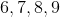Then we can count up.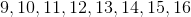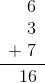### Example Question #82 : Representing And Solving Addition And Subtraction Problems

Lauren haspencils,markers, andnotebooks in her backpack. How many total items does Lauren have in her backpack?Explanation:

This is an addition problem because we want to know how many total supplies Lauren has in her backpack when we put everything together. When we put things together we are adding.

We can start atand count up.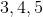Then we can count up.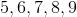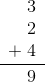1 2 3 4 5 6 7 9 Next →

### All Common Core: 1st Grade Math Resources